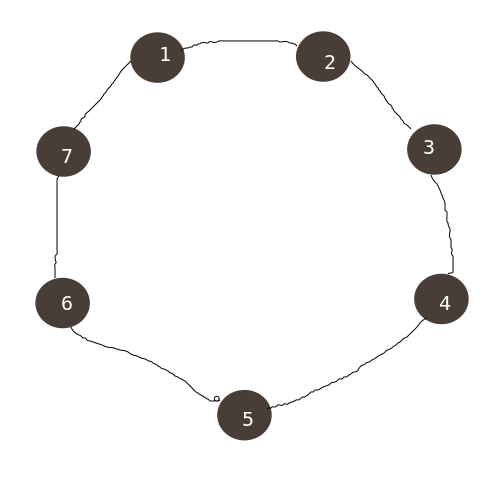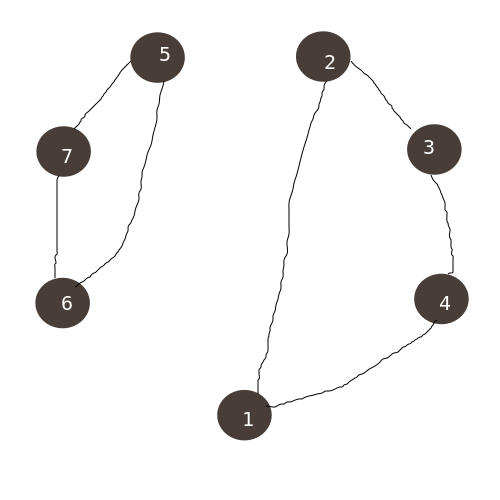# 博客

## #104. Counting Swaps 题解

2018-03-08 18:16:44 By poorpool#include <iostream>
#include <cstring>
#include <cstdio>
using namespace std;
typedef long long ll;
int n, uu, hea, cnt, bel, din, hav, ans, T;
int jie;
struct Edge{
int too, nxt;
}edge;
const int mod=1e9+9;
void add_edge(int fro, int too){
edge[++cnt].nxt = hea[fro];
edge[cnt].too = too;
hea[fro] = cnt;
}
void dfs(int x, int c){
bel[x] = c;
for(int i=hea[x]; i; i=edge[i].nxt){
int t=edge[i].too;
if(!bel[t])
dfs(t, c);
}
}
int ksm(int a, int b){
if(!a)    return 1;
if(b<0)    return 1;
int re=1;
while(b){
if(b&1)    re = ((ll)re * a) % mod;
a = ((ll)a * a) % mod;
b >>= 1;
}
return re;
}
int main(){
cin>>T;
jie = 1;
for(int i=1; i<=100000; i++)
jie[i] = ((ll)jie[i-1] * i) % mod;
while(T--){
memset(hav, 0, sizeof(hav));
memset(bel, 0, sizeof(bel));
memset(hea, 0, sizeof(hea));
cnt = din = 0;
scanf("%d", &n);
for(int i=1; i<=n; i++){
scanf("%d", &uu);
}
for(int i=1; i<=n; i++)
if(!bel[i])
dfs(i, ++din);
for(int i=1; i<=n; i++)
hav[bel[i]]++;
ans = 1;
for(int i=1; i<=din; i++)
ans = ((ll)ans * ksm(hav[i], hav[i]-2)) % mod;
ans = ((ll)ans * jie[n-din]) % mod;
for(int i=1; i<=din; i++)
ans = ((ll)ans * ksm(jie[hav[i]-1], mod-2)) % mod;
cout<<ans<<endl;
}
return 0;
}